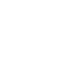# Widget Calculator

## The widget allows you to automatically calculate the data in the fields according to a given formula and display the result in the selected field.

from 16 \$ per month
Demo period for 7 days
Widget setup
How to set up and use:
Go to the "Settings" tab (to the right of "Description") within this widget and click the "Add formula" button
Specify the name of the formula, assign the order of calculation, and also write your formula.
To substitute values ​​from the field into the formula, insert "@" and select from the proposed list.
As part of the formula setup, you can specify the formula execution conditions under which this formula will work.
In the "Reference" section, you can add numerical values ​​to the selected values ​​in the fields of the list / multi-list / flag / radio button type
To substitute values ​​in the formula based on the directory, insert "#" and select from the proposed list
Any mathematical formulas are supported
With the widget you can:
Customize the rounding format you need
Substitute the values ​​of numeric fields in the formula, as well as use list fields in calculations
Set the calculation order if you use several consecutive calculations
Make calculations using any mathematical formulas and substitute the calculation result in the required field
Set up calculation conditions
With the help of the maximum flexible setting of conditions, you can:
Check for the presence of a specified value in a specific field, or a value from another field
Set up a condition for the formula to run only in cases where the deal is in a certain status (or funnel), or has certain responsible persons
Check whether a transaction has goods or categories of goods
Assign possible items in list fields (list, multi-box, flag, radio button) numerical values ​​and use them in calculations
Use List Field Values ​​in a Calculation
Calculate with a trigger in Digital Pipeline
The widget allows you to perform automatic calculation not only with manual entry, but also when a transaction reaches a certain status (or event)
In the trigger settings you can choose which formulas to run
Cost of use
6
months
120 \$
20 \$ / month
9
months
162 \$
18 \$ / month
Popular
12
months
192 \$
16 \$ / month
Profitable
10% discount
20% discount
Need development for Kommo?
If you need functionality that is not in ready-made solutions, we can develop it